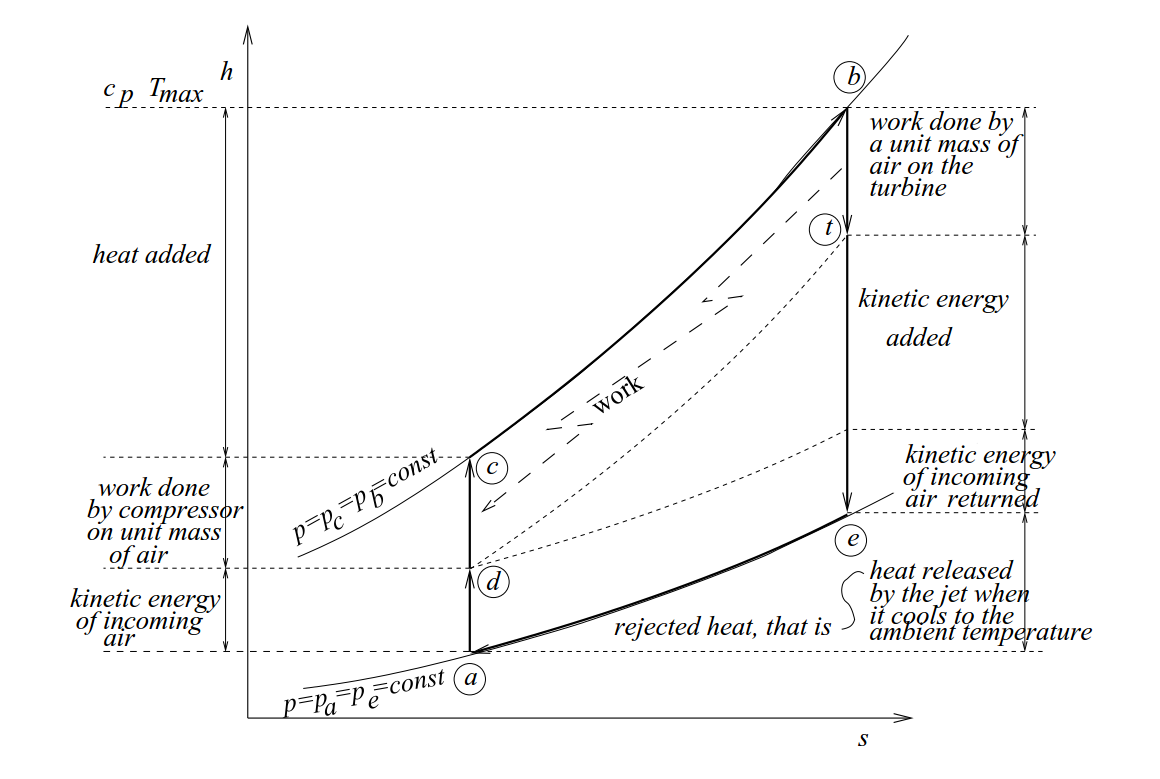# TurboJet h-s diagram comparison

This is a turbojet h-s (T vs s/cp plotted in fact) diagram comparison tool.Note the relationship between the elements of the diagram and the engine parameters such as specific thrust and efficiencies. Thermal efficiency equals the ratio of the kinetic energy added and the added heat. Propulsion efficiency grows when the ratio of the kinetic energy of incoming air to the kinetic energy in the exhaust jet increases. Kinetic energy of the exhaust jet is the sum of the kinetic energy added and the kinetic energy returned. Specific thrust is proportional to the difference between the square root of the kinetic energy in the exhaust jet and the square root of the kinetic energy of the incoming air.

Change the design parameters of the engine, and, by comparing the diagrams, see how the difference in the design parameters affects the engine performance.

(The tool does not work under IE9: use other browsers.)

M = Ta= T0b= prc = ηd= ηc =ηt= ηn = rb =

M = Ta= T0b= prc = ηd= ηc =ηt= ηn = rb =